#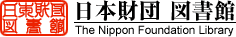ANALYSIS OF DISSOLVED OXYGEN AND TRANSPORT OF FISH EGGS IN A BAY
Yoshihiro Suenaga1, Koichi Masuda2, Takashi Sasaki1, Hee-Do Ahn3, Tetsuro Kobayashi1, Takashi Hoshino4 and Kaori Yasuoka4

1Faculty of Engineering, Kagawa University
Takamatsu, Kagawa Pref., JAPAN
suenaga@eng.kagawa-u.ac.jp

2College of Science and Technology, Nihon University
Chiba, JAPAN

3Korea Ocean Research and Development Institute
Seoul, KOREA

4Kuroshio Marine Technology, Co, LTD
JAPAN

ABSTRACT

Coastal aquaculture has been increased and developed in a semi-enclosed bay. In Seto inland Sea, Japan could produce marine aquaculture productions to supply the population demands. While the feeding rate of fish farming in Sido Bay, Kagawa Pref., Japan is approximately 12,378 tons a year, with the highest rate in summer. Therefore, the pollution from floating aquaculture cage can affect the environment via fecal waste, dissolved waste and excess feed settles. After the field experiments, it was found the tidal current, bottom topography and DO consumption rate by sediment are important factors to evaluate the canying capacity of aquaculture grounds. The authors proposed a more appropriate evaluation method for DO by using three-dimensional numerical model taking the effect of oxygen consumption by the fish in the aquaculture cage and bottom sediment into consideration. In this study has revealed that transportation of fish eggs and larvae from spawning ground to the nursery ground in and around the semi-enclosed bay by using numerical simulation model. Then, it is necessary to take the system for creating fishery ground and to estimate the carrying capacity of aquaculture grounds.

INTRODUCTION

Sido Bay is a small coastal aquaculture system that located 34°20'N and 134°11'E. The climate in this region is influence by the tidal current, the Seto Inland Sea Japan. The bay is about 6 km in length and approximately 4 km in width at the mouth with a surface area of ca. 20 km2 average depth of 8.3 m and the total water volume of ca. 140 m3 (Fig.1). Recently variable environmental problems have been caused by red tide, such as poor oxygen concentration at the bottom, exploitation of sand resources for construction materials and many kinds of coastal developments. Although aquaculture habitats in the Sido bay have been decreased, the impact of aquaculture on environment could be manifest in a wide variety of ways (i.e. waste feed setting and oxygen deplete). We have conducted field research to confirm the oxygen consumption by the fish in the aquaculture cage and bottom sediment by using undisturbed core method. In this paper, we described a more appropriate evaluation method for the carrying capacity of aquaculture grounds by using three-dimensional numerical simulation models (Suenaga et al., 1996; Fujihara et al., 1997) taking the effect of oxygen consumption rate at each area in Sido bay into consideration. Also, the authors calculated the transport of fish eggs and larvae in and around the Bisan-Seto area to clarify the nursery ground by using Euler-Lagrangian method. This method is useful in estimating the carrying capacity for aquaculture grounds in a semi-enclosed bay.

 （Enlarge: 64KB）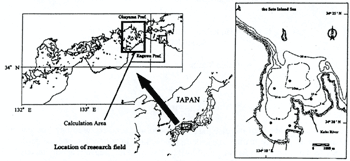Figure 1. Bottom topography of Sido bay
●Collection of water and sediment samples

MATERIALS & METHODS

Governing equations

The current in the Sido bay is under the influence of tidal current and west and east component of current is predominant along the both shores of Okayama Prefecture and Kagawa Prefecture. A multi-level model (Suenaga et al., 1996; Fujihara et al., 1997; Suenaga et al., 1999; Sakuta et al., 1993) presently employed is represented by the momentum equation on the rotating earth accepting f-plane and hydrostatic approximations, the continuity equation, equation of free surface, the diffusion equations of water temperature and salinity and DO and state equation, as follows (Eqs. (1)-(8));

 （Enlarge: 5KB）（Enlarge: 11KB）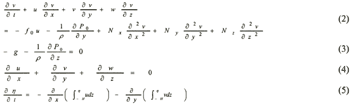For T

 （Enlarge: 6KB）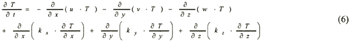For S and DO

 （Enlarge: 8KB）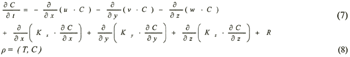where u and v are the horizontal current vectors, w the vertical current velocity, T the water temperature, C is the state variables (the salinity, S and DO), ρ the density of water, g the acceleration due to gravity, f (=8.15 × 10-5 /s: at 34°N) the Coliolis parameter, η the elevation from the mean sea level (positive upward), H the water depth, Nx, Ny (=0.01 m2/s) and Nz {friction coefficient at surface (=0.0013): bottom (=0.0026): internal (=0.0001)} the horizontal and vertical eddy viscosity coefficients, kx (=104cm/s) and ky (=1 cm2/s) the horizontal and vertical turbulent diffusion coefficients of water temperature, Kx , Ky (=104 cm2/s) and Kz (= 1 cm2/s) the horizontal and vertical diffusion coefficients of salinity and k the unit vector(positive upward), respectively (Sasaki and Inoue, 1984).

In the diffusion equation of DO, the last term "R" in equation (7) includes the DO flux by water surface (Sasaki and Inoue, 1984). Re-aeration, consumption rate (A:0.003, B:0.004. C:0.005, D:0.008, ml/sec/m2) by the respiration of fish (yellowtail fish), consumption by suspended matters (0.068ml/l/hr) and sediment. Also, the consumption rate by sediment (A:0.017, B:0.017, C:0.018, D:0.018, ml/sec/m2) was measured by undisturbed core method.

Euler-Lagrangian Method

The eggs and larvae treated as particles were traced in the computed flow field for their planktonic period (27 days) using the Euler-Lagrangian Method. The governing equations are as follows:

 dX/dt=V (9) X(0)=X0 (10) X(n+1)*=X(n)+F(n)Δt (11) X(n+1)=X(n)+(F(n)+F(n+1)*)/2*Δt (12) F(n)=V(n)+{(V(n)・∇)V(n)}Δt (13)

where X is the position, V the velocity, ∇ the horizontal differential operation, Δt (=20s) the time increment; superscript means the time level and * is temporary value at the time level. Initial value problem of the differential equations expressed in equations (9) and (10) is solved using two-step Runge-Kutta integration method expressed in equations (11)-(13). The velocity and shear at the egg/larva position are calculated by interpolation using surrounding values. Particles are located the depth of 10 m and transported only by water movement for the first 3 days and then gradually swim up to the depth of 3 m for 12 hours (Nakata et. al., 1999). After that they gradually swim down to depth of 10 m for 12 hours, and they repeat that movement during 27 days.

Computed domain

Figure 2 shows the computed domain of this study area. The bottom topography in and around the Western Bisan-Seto area and computational domain are shown in Figure 2. The whole body of water is described into 500 m × 500 m square meshes horizontally and three levels vertically (1st level:0-6m, 2nd level:6-15 m, 3rd level:15 m-bottom) for the computation. Tidal flow (tidal level: half amplitude=1 m) is given at the each entrance of Bisan-Seto area as a boundary condition. The flow fields were first reproduced using a multi-level density flow model with observed velocities as boundary tidal conditions. In Sido Bay, the whole body of water is described into 100 m × 100 m square meshes horizontally and six levels vertically (1st:O-1.5 m, 2nd:1.5-3.5 m, 3rd:3.5-6.5 m, 4th:6.5-9.5 m, 5th:9.5-25.5 m, 6th:25.5 m-bottom) for the computation. Tidal flow is given at the each entrance of Sido bay as boundary conditions shown in Table 1. Also, river discharge was added from Kabe-river (3.9 × 104 m3/day) to the sea (Fig.1).

Table 1. The boundary conditions for calculation
 Area Amplitude (m) Period (hr) Phase lag (deg) A 0.563 12.42 324.5 A' 0.563 12.42 324.5 B 0.456 12.42 331.9 B' 0.456 12.42 331.9

 （Enlarge: 71KB）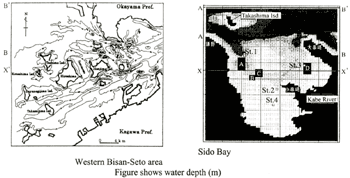Figure 2. Computed domain of each study fields